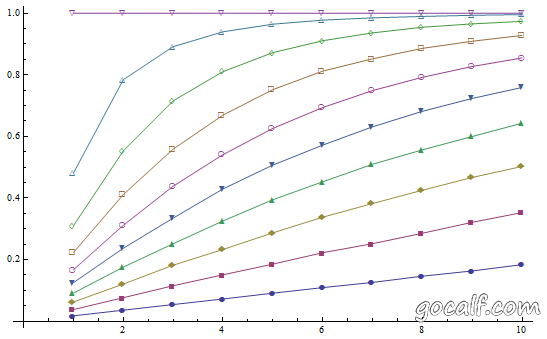1 2 3 4 5 6 7 8 9 10 11 12 13 from random import Random def WeightedRandomSelect(rand=None): selection = None totalweight = 0.0 if rand is None: rand = Random() while True: # Outputs the current selection and gets next item (item, weight) = yield selection totalweight += weight if rand.random() * totalweight < weight: selection = item 

\begin{equation*} \frac{w_i}{w}=\frac{w_i}{\sum_{k=1}^{i}{w_k}} \end{equation*}

\begin{equation*} \frac{w-w_j}{w}=\frac{\sum_{k=1}^{j-1}{w_k}}{\sum_{k=1}^{j}{w_k}} \end{equation*}

\begin{equation*} p_i=\frac{w_i}{\sum_{k=1}^{i}{w_k}}\times\frac{\sum_{k=1}^{i}{w_k}}{\sum_{k=1}^{i+1}{w_k}}\times\frac{\sum_{k=1}^{i+1}{w_k}}{\sum_{k=1}^{i+2}{w_k}}\times\cdots\times\frac{\sum_{k=1}^{n-1}{w_k}}{\sum_{k=1}^{n}{w_k}}=\frac{w_i}{\sum_{k=1}^{n}{w_k}} \end{equation*}

  1 2 3 4 5 6 7 8 9 10 11 12 13 14 15 16 # Sample code to use WeightedRandomSelect function # Use an arithmetic sequence as weights n = 10 # weights are [1, 2, 3, ..., 10] weights = [i + 1 for i in xrange(n)] repeat = 100000 occurrences = [0 for i in xrange(n)] rand = Random() for i in xrange(repeat): selector = WeightedRandomSelect(rand) selector.next() selection = None for item in xrange(n): selection = selector.send((item, weights[item])) occurrences[selection] += 1 print occurrences 

[1723, 3644, 5405, 7326, 9027, 10903, 12678, 14784, 16345, 18165]


1 : 2 : 3 : 4 : 5 : 6 : 7 : 8 : 9 : 10
= 0.0181818 : 0.0363636 : 0.0545455 : 0.0727273 : 0.0909091 : 0.109091 : 0.127273 : 0.145455 : 0.163636 : 0.181818


## 扩展：选取 m 个元素，概率理论值

\begin{equation*} p_i(2)=\frac{w_i}{w}+\sum_{j\neq i}\left(\frac{w_j}{w}\times\frac{w_i}{w-w_j}\right) \end{equation*}

\begin{equation*} \bar p_i(2)=\sum_{j\neq i}\left(\frac{w_j}{w}\times\frac{w-w_j-w_i}{w-w_j}\right) \end{equation*}

\begin{equation*} \bar p_i(m)=\sum_{j_1}\left(\frac{w_{j_1}}{w}\sum_{j_2}\left(\frac{w_{j_2}}{w-w_{j_1}}\sum_{j_3}\left(\frac{w_{j_2}}{w-w_{j_1}-w_{j_2}}\cdots\sum_{j_m}\frac{w_{j_m}}{w-\sum_{k=1}^{m-1}w_{j_k}}\right)\right)\right) \end{equation*}

  1 2 3 4 5 6 7 8 9 10 11 12 13 14 15 16 17 18 def Foo(weights, ids, totalweight, m, i, times): if times == m: return 1 p = 0.0 for j in ids: ids.remove(j) p += float(weights[j]) / totalweight \ * Foo(weights, ids, totalweight - weights[j], m, i, times + 1) ids.add(j) return p def CalcSampleProbability(weights, m, i): n = len(weights) assert 0 <= i < n, 'invalid i' assert 0 < m <= n, 'invalid m' ids = set(xrange(n)) ids.remove(i) p = Foo(weights, ids, sum(weights), m, i, 0) return 1 - p 

Mathematica 提供了 RandomSample 函数，支持带权选取，当然它是在遍历之前就已经知道元素个数的。给它一组等差分布的权重，可以看出十万次随机选取后得到的概率分布与上面的理论分布非常接近。

count = 10;
weights = Range[count];
elems = Range[count];
retry = 100000;
map = Table[
freq = ConstantArray[0, count];
For [i = 0, i < retry, i++,
freq += BinCounts[RandomSample[weights -> elems, m], {1, count + 1, 1}]
];
freq, {m, 1, count, 1}
];
ListLinePlot[map / retry, PlotMarkers -> Automatic]Mathematica RandomSample 随机选取 m 个元素，第 i 个元素被选中的概率

So what do you think? Did I miss something? Is any part unclear? Leave your comments below.